# Electrons and Wave-Particle DualityPage 1

#### WATCH ALL SLIDES

Slide 1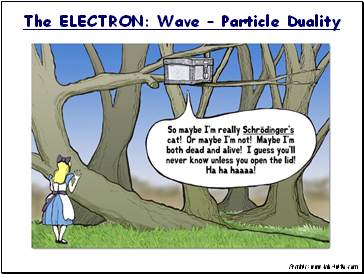The ELECTRON: Wave – Particle Duality

Graphic: www.lab-initio.com

Slide 2## The Dilemma of the Atom

Electrons outside the nucleus are attracted to the protons in the nucleus

Charged particles moving in curved paths lose energy

What keeps the atom from collapsing?

Slide 3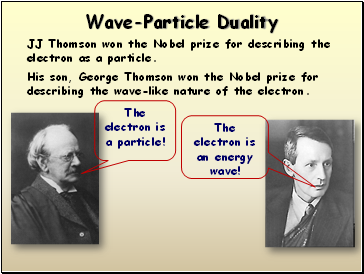## Wave-Particle Duality

JJ Thomson won the Nobel prize for describing the electron as a particle.

His son, George Thomson won the Nobel prize for describing the wave-like nature of the electron.

The electron is a particle!

The electron is an energy wave!

Slide 4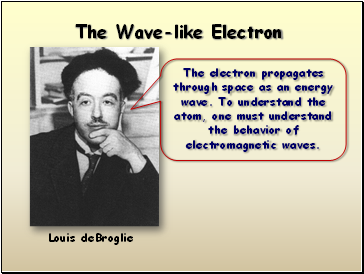## The Wave-like Electron

Louis deBroglie

The electron propagates through space as an energy wave. To understand the atom, one must understand the behavior of electromagnetic waves.

Slide 5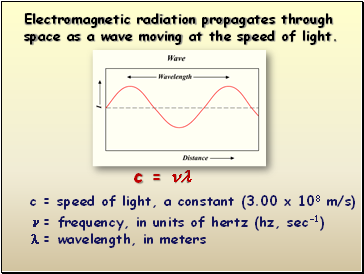c = 

c = speed of light, a constant (3.00 x 108 m/s)

 = frequency, in units of hertz (hz, sec-1)

 = wavelength, in meters

Electromagnetic radiation propagates through space as a wave moving at the speed of light.

Slide 6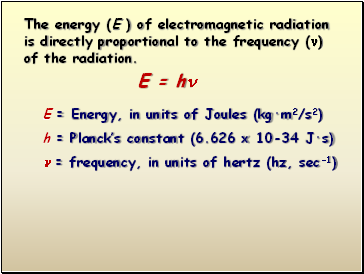E = h

E = Energy, in units of Joules (kg·m2/s2)

h = Planck’s constant (6.626 x 10-34 J·s)

 = frequency, in units of hertz (hz, sec-1)

The energy (E ) of electromagnetic radiation is directly proportional to the frequency () of the radiation.

Slide 7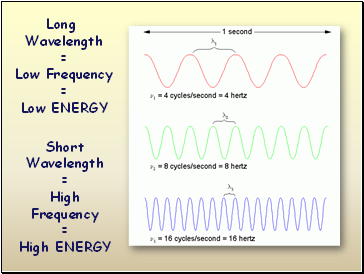Long

Wavelength

=

Low Frequency

=

Low ENERGY

Short

Wavelength

=

High Frequency

=

High ENERGY

Wavelength Table

Slide 8## Answering the Dilemma of the Atom

Treat electrons as waves

As the electron moves toward the nucleus, the wavelength shortens

Shorter wavelength = higher energy

Higher energy = greater distance from the nucleus

Slide 9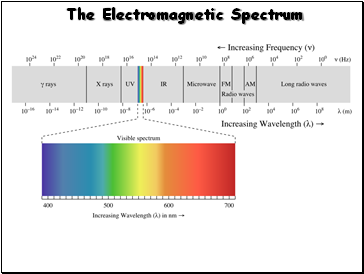## The Electromagnetic Spectrum

Slide 10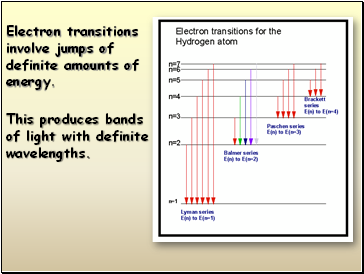This produces bands

of light with definite wavelengths.

Electron transitions involve jumps of definite amounts of energy.

Slide 11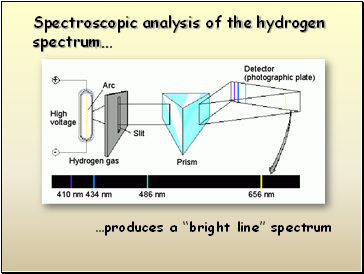…produces a “bright line” spectrum

Go to page:
1  2